September 1, 2020

# How to Use If-Else Statements and Loops in R

When we're programming in R (or any other language, for that matter), we often want to control when and how particular parts of our code are executed. We can do that using control structures like if-else statements, for loops, and while loops.

Control structures are blocks of code that determine how other sections of code are executed based on specified parameters. You can think of these as a bit like the instructions a parent might give a child before leaving the house:

"If I'm not home by 8pm, make yourself dinner."

Control structures set a condition and tell R what to do when that condition is met or not met. And unlike some kids, R will always do what we tell it to! You can learn more about control structures in the R documentation if you would like.

In this tutorial, we assume you’re familiar with basic data structures, and arithmetic operations in R.

Not quite there yet? Check out our Introductory R Programming course that's part of our Data Analyst in R path. It’s free to start learning, there are no prerequisites, and there's nothing to install — you can start learning in your browser right now.

(This tutorial is based on our intermediate R programming course, so check that out as well! It's interactive and will allow you to write and run code right in your browser.)

## Comparison Operators in R

In order to use control structures, we need to create statements that will turn out to be either `TRUE` or `FALSE`. In the kids example above, the statement "It's 8pm. Are my parents home yet?" yields `TRUE` ("Yes") or `FALSE` ("No"). In R, the most fundamental way to evaluate something as `TRUE` or `FALSE` is through comparison operators.

Below are six essential comparison operators for working with control structures in R:

• `==` means equality. The statement `x == a` framed as a question means "Does the value of `x` equal the value of `a`?"
• `!=` means "not equal". The statement `x == b` means "Does the value of `x` not equal the value of `b`?"
• `<` means "less than". The statement `x < c` means "Is the value of `x` less than the value of `c`?"
• `<=` means "less than or equal". The statement `x <= d` means "Is the value of `x` less or equal to the value of `d`?"
• `>` means "greater than". The statement `x >` e means "Is the value of `x` greater than the value of `e`?"
• `>=` means "greater than or equal". The statement `x >= f` means "Is the value of `x` greater than or equal to the value of `f`?"

## Understanding If-Else in R

Let's say we're watching a sports match that decides which team makes the playoffs. We could visualize the possible outcomes using this tree chart: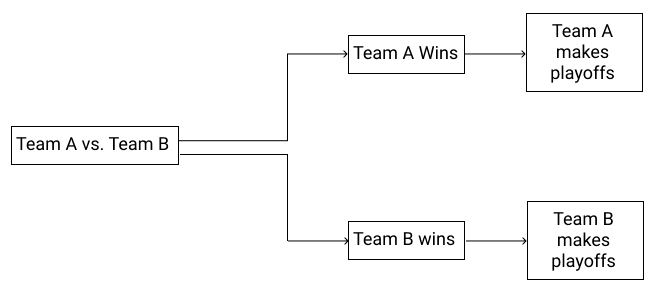As we can see in the tree chart, there are only two possible outcomes. If Team A wins, they go to the playoffs. If Team B wins, then they go.

Let's start by trying to represent this scenario in R. We can use an if statement to write a program that prints out the winning team.

If statements tell R to run a line of code if a condition returns `TRUE`. An if statement is a good choice here because it allows us to control which statement is printed depending on which outcome occurs.

The figure below shows a conditional flow chart and the basic syntax for an if statement: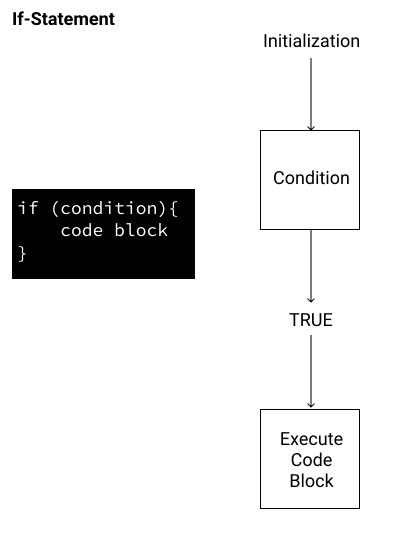Our if statement's condition should be an expression that evaluates to `TRUE` or `FALSE`. If the expression returns TRUE, then the program will execute all code between the brackets `{ }`. If FALSE, then no code will be executed.

Knowing this, let's look at an example of an if statement that prints the name of the team that won.

``````team_A <- 3 # Number of goals scored by Team A
team_B <- 1 # Number of goals scored by Team B
if (team_A > team_B){
print ("Team A wins")
}``````
``````"Team A wins"
``````

It worked! Because Team A had more goals than Team B, our conditional statement(`team_A > team_B`) evaluates to `TRUE`, so the code block below it runs, printing the news that Team A won the match.

## Adding the else Statement in R

In the previous exercise, we printed the name of the team that will make the playoffs based on our expression. Let's look at a new matchup of scores. What if Team A had 1 goal and Team B had 3 goals. Our team_A > team_B conditional would evaluate to `FALSE`. As a result, if we ran our code, nothing would be printed. Because the if statement evaluates to false, the code block inside the if statement is not executed:

``````team_A <- 1 # Number of goals scored by Team A
team_B <- 3 # Number of goals scored by Team B
if (team_A > team_B){
print ("Team A will make the playoffs")
}
``````

If we return to our original flow chart, we can see that we've only coded a branch for one of the two possibilities: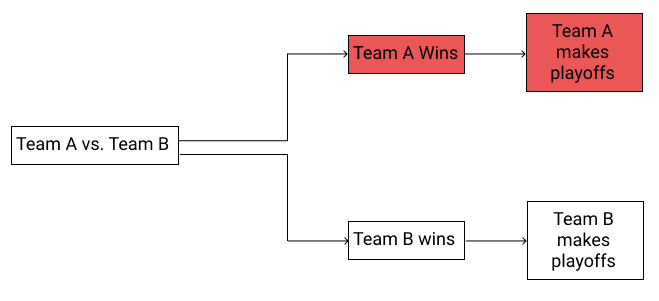Ideally, we'd like to make our program account for both possibilities and "Team B will make the playoffs" if the expression evaluates to FALSE. In other words, we want to be able to handle both conditional branches: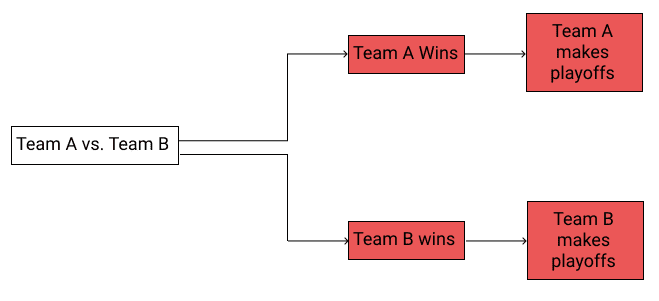To do this, we'll add an else statement to turn this into what's often called an if-else statement. In R, an if-else statement tells the program to run one block of code if the conditional statement is `TRUE`, and a different block of code if it is `FALSE`. Here's a visual representation of how this works, both in flowchart form and in terms of the R syntax:

******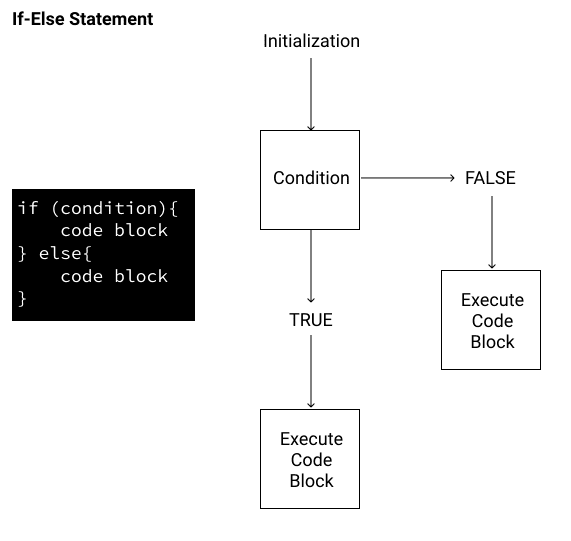**

To generalize, if-else in R needs three arguments:

1. A statement (e.g. comparison operator) that evaluates to TRUE or FALSE.
2. The value that R should return if the comparison operator is TRUE.
3. The value that R should return if the comparison operator is FALSE.

So for our example we need to add a block of code that runs if our conditional expression `team_A > team_B` returns `FALSE`. We can do this by adding an `else` statement in R. If our comparison operator evaluates to FALSE, let's print "Team B will make the playoffs."

``````
team_A <- 1 # Number of goals scored by Team A
team_B <- 3# Number of goals scored by Team B
if (team_A > team_B){
print ("Team A will make the playoffs")
} else {
print ("Team B will make the playoffs")
}
``````
``````"Team B will make the playoffs"
``````

To recap:

• The essential characteristic of the if statement is that it helps us create a branching path in our code.
• Both the `if` and the `else` keywords in R are followed by curly brackets `{ }`, which define code blocks.
• Each of the code blocks represent one of the paths shown in the diagram.
• R does not run both, and it uses the comparison operator to decide which code block to run.

## Moving Beyond Two Branches

So far, we've worked under the assumption that each of the decisions in our control structure had only two branches: one corresponding to `TRUE` and another to `FALSE`. There are plenty of occasions where we have more than two since some decisions don't boil down to a "Yes" vs "No".

Suppose, for a moment, that we are watching a sports match that can end in a tie. The control structure from our last example does not account for this. Fortunately, R provides a way to incorporate more than two branches in an `if` statement with the `else if` keyword. The else if keyword provides another code block to use in an `if` statement, and we can have as many as we see fit. Here's how this would look:

``````
team_A <- 2 # Number of goals scored by Team A
team_B <- 2# Number of goals scored by Team B
if (team_A > team_B){
print ("Team A won")
} else if (team_A < team_B){
print ("Team B won")
} else {
"Team A & B tied"
}
``````
``````"Team A & B tied"
``````

Each potential game outcome gets its own branch. The `else` code block helps cover us for any situation where there is a tie.

## Using the for loop in R

Now that we've used if-else in R to display the results of one match, what if we wanted to find the results of multiple matches? Let's say we have a list of vectors containing the results of our match: `matches <- list(c(2,1),c(5,2),c(6,3))`.

Keep in mind that we'll have to use `[[]]` when indexing, since we want to return a single value within each list on our list, not the value with the list object. Indexing with `[]` will return a list object, not the value.

So, for example, in the code we have above, `matches[]` is calling the first index of the second list (i.e., Team A's score in Game 2).

Assuming that Team A's goals are listed first (the first index of the vector) and Team B's are second, we could find the results using if-else in R like this:

``````
if (matches[] > matches[]){
print ("Win")
} else {
print ("Loss")
}

if (matches[] > matches[]){
print ("Win")
} else {
print ("Loss")
}

if (matches[] > matches[]){
print ("Win")
} else {
print ("Loss")
}
``````

And this would print:

``````"Win"
"Win"
"Win"
``````

This code works, but if we look at this approach it's easy to see a problem. Writing this out for three games is already cumbersome. What if we had a list of 100 or 1000 games to evaluate?

We can improve on our code by performing the same action using a for loop in R. A for loop repeats a chunk of code multiple times for each element within an object. This allows us to write less code (which means less possibility for mistakes) and it can express our intent better. Here's a flow chart representation, and the syntax in R (which looks very similar to the if syntax).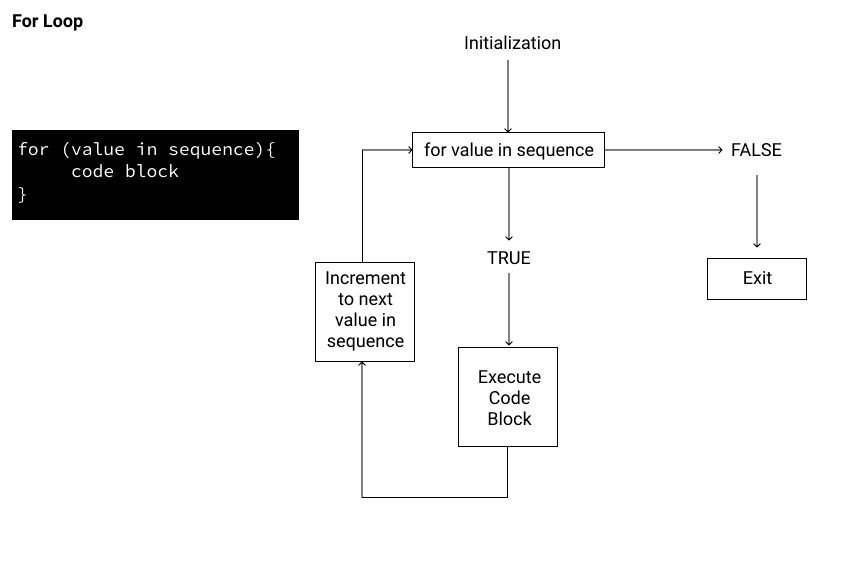In this diagram, for each value in the sequence, the loop will execute the code block. When there are no more values left in the sequence, this will return `FALSE` and exit the loop.

Let's break down what's going on here.

• sequence: This is a set of objects. For example, this could be a vector of numbers c(1,2,3,4,5).
• value: This is an iterator variable you use to refer to each value in the sequence. See variables naming conventions in the first course for valid variable names.
• code block: This is the expression that's evaluated.

Let's look at a concrete example. We'll write a quick loop that prints the value of items in a list, and we'll create a short list with two items: Team A and Team B.

``````
teams <- c("team_A","team_B")
for (value in teams){
print(value)
}
``````
``````"team_A"
"team_B"
``````

Since `teams` has two values, our loop will run twice. Here's a visual representation of what's going on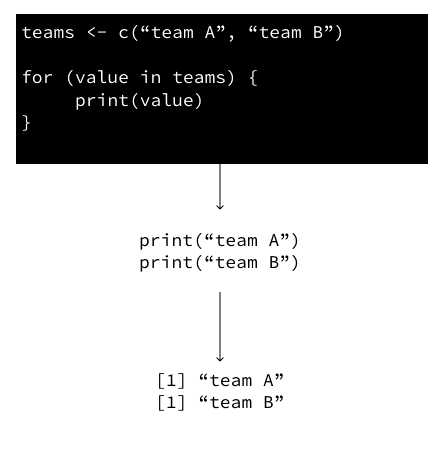Once the loop displays the result from the first iteration, the loop will look at the next value in the position. As a result, it'll go through another iteration. Since there aren't any more values in the sequence, the loop will exit after "team_B".

In aggregate, the final result will look like this:

``````"team_A"
"team_B"
``````

## Adding the Results of a Loop to an Object in R

Now that we've written out our loop, we'll want to store each result of each iteration in our loop. In this post, we'll store our values in a vector, since we're dealing with a single data type.

As you may already know from our R Fundamentals course, we can combine vectors using the `c()` function. We'll use the same method to store the results of our for loop.

``````
for (match in matches){
print(match)
}
``````

Now, let's say we wanted to get the total goals scored in a game and store them in the vector. The first step we'd need to do would be to add each score from our list of lists together, which we can do using the `sum()` function. We'll have our code loop through `matches` to calculate the sum of the goals in each match.

``````
matches <- list(c(2,1),c(5,2),c(6,3))
for (match in matches){
sum(match)
}``````

But we still haven't actually saved those goal totals anywhere! If we want to save the total goals for each match, we can initialize a new vector and then append each additional calculation onto that vector, like so:

``````
matches <- list(c(2,1),c(5,2),c(6,3))
total_goals <- c()
for (match in matches){
total_goals <- c(total_goals, sum(match))
}``````

## Using if-else Statements Within for loops in R

Now that we've learned about if-else in R, and for loops in R, we can take things to the next level and use if-else statements within our for loops to give us the results of multiple matches.

To combine two control structures, we'll place one control structure in between the brackets `{ }` of another.

``````matches <- list(c(2,1),c(5,2),c(6,3))
``````

Then we'll create a for loop to loop through it:

``````for (match in matches){
}
``````

This time, rather than print our results, let's add an if-else statement into the for loop.

In our scenario, we want our program to print whether Team A won or lost the game. Assuming Team A's goals is the first of each pair of values and the opponents is the second index, we'll need to use a comparison operator to compare the values. After we make this comparison, if team_A's score is higher, we'll print "Win". If not, we'll print "Lose".

When indexing into the iterable variable match, we can use either `[]` or `[[]]` since the iterable is a vector, not a list.

``````
matches <- list(c(2,1),c(5,2),c(6,3))
for (match in matches){
if (match > match){
print("Win")
} else {
print ("Lose")
}
}
``````
``````"Win"
"Win"
"Win"
``````

## Breaking the for loop in R

Now that we've added an if-else statement, let's look at how to stop a for loop in R based on a certain condition. In our case, we can use a `break` statement to stop the loop as soon as we see Team A has won a game.

Using the for loop we wrote above, we can insert the break statement inside our if-else statement.

``````
matches <- list(c(2,1),c(5,2),c(6,3))
for (match in matches){
if (match > match){
print("Win")
break
} else {
print("Lose")
}
}``````
``````"Win"
``````

## Using a while loop in R

In the previous exercise, we used a for loop in R to repeat a chunk of code that gave us the result of the match. Now that we've returned the results of each match, what if we wanted to count the number of wins to determine if they make the playoffs? One method of returning the results for the first four games is to use a while loop in R.

A while loop in R is a close cousin of the for loop in R. However, a while loop will check a logical condition, and keep running the loop as long as the condition is true. Here's what the syntax of a while loop looks like:

``````while(condition){
expression
}``````

In flow-chart form: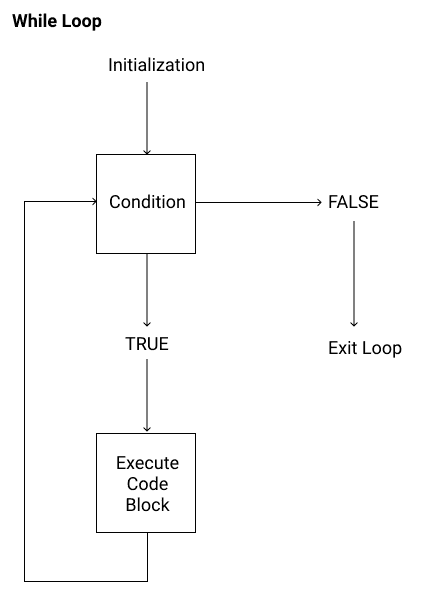If the condition in the while loop in R is always true, the while loop will be an infinite loop, and our program will never stop running. This is something we definitely want to avoid! When writing a while loop in R, we want to ensure that at some point the condition will be false so the loop can stop running.

Let's take a team that's starting the season with zero wins. They'll need to win 10 matches to make the playoffs. We can write a while loop to tell us whether the team makes the playoffs:

``````wins <- 0
while (wins < 10){
print ("Does not make playoffs")
wins <- wins + 1
}``````
``````"Does not make playoffs"
"Does not make playoffs"
"Does not make playoffs"
"Does not make playoffs"
"Does not make playoffs"
"Does not make playoffs"
"Does not make playoffs"
"Does not make playoffs"
"Does not make playoffs"
"Does not make playoffs"
``````

Our loop will stop running when wins hits 10. Notice, that we continuously add 1 to the win total, so eventually, the win < 10 condition will return `FALSE`. As a result, the loop exits.

Don't worry if this whole process seems daunting, while loops in R take time to understand, but they are powerful tools once mastered. There are a lot of different variables to juggle, but the key to understanding the while loop is to know how these variables change every time the loop runs.

Let's write our first while loop in R, counting Team A wins!

## Using an if-else Statement within a while loop in R

Now that we've printed the status of the team when they don't have enough wins, we'll add a feature that indicates when they do make the playoffs.

To do this, we'll need to add an if-else statement into our while loop. Adding an if-else statement into a while loop is the same as adding it to a for loop in R, which we've already done. Returning to our scenario where 10 wins allows Team A to make the playoffs, let's add an if-else conditional.

The if-else conditional will go between the brackets of the while loop, in the same place we put it into the for loop earlier.

``````
wins <- 0
while (wins <= 10){
if (wins < 10){
print("does not make playoffs")
} else {
print ("makes playoffs")
}
wins <- wins + 1
}``````
``````"does not make playoffs"
"does not make playoffs"
"does not make playoffs"
"does not make playoffs"
"does not make playoffs"
"does not make playoffs"
"does not make playoffs"
"does not make playoffs"
"does not make playoffs"
"makes playoffs"
``````

## Breaking the while loop in R

Let's say the maximum number of wins a team can have in a season is 15. To make the playoffs, we'll still need 10 wins, so we can end our loop as soon as Team A has hit this number.

To do this, we can use another `break` statement. Again, this functions the same way in a while loop that it does in a for loop; once the condition is met and `break` is executed, the loop ends.

``````
wins <- 0
playoffs <- c()
while (wins <= 15){
if (wins < 10){
print("does not make playoffs")
playoffs <- c(playoffs, "does not make playoffs")
} else {
print ("makes playoffs")
playoffs <- c(playoffs, "makes playoffs")
break
}
wins <- wins + 1
}``````
``````"does not make playoffs"
"does not make playoffs"
"does not make playoffs"
"does not make playoffs"
"does not make playoffs"
"does not make playoffs"
"does not make playoffs"
"does not make playoffs"
"does not make playoffs"
"does not make playoffs"
"makes playoffs"
``````

## Intuition Behind the while loop

The for loop in R is the loop that you'll probably deal with the most often. But the while loop is still useful to know about.

To distinguish between these two types of loops, it's useful to think of a for loop as dealing with a chore list. The idea is that you have a set amount of chores to finish, and once you do all of your chores, you're done. The key here is that there is a set amount of items that we need to loop through in a for loop.

On the other hand, a while loop is like trying to reach a milestone, like raising a target amount of money for a charity event. For charity events, you typically perform and do things to raise money for your cause, like running laps or giving services to people. You do these tasks until you reach your target goal, and it's not clear from the beginning how many tasks you need to do to reach the goal. That's the key idea behind a while loop: repeat some actions (read: a code chunk) until a condition or goal is met.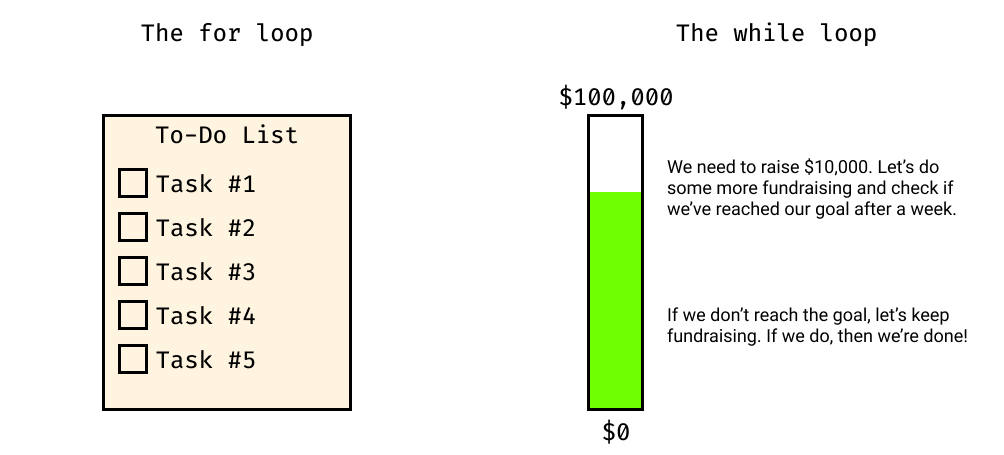While loops play a major role in heavy analytical tasks like simulation and optimization. Optimization is the act of looking for a set of parameters that either maximize or minimize some goal.

In other data analysis tasks, like cleaning data or calculating statistics, while loops are not so useful. These tasks form the brunt of what you encounter in the Data Analyst in R path and perhaps your career, but it's always good to know what tools are available to you as a programmer.

## Next Steps

In this tutorial, we've developed a basic if statement into a more complex program that executes blocks of code based on logical conditions.

These concepts are important aspects of R programming, and they will help you write significantly more powerful code. But we're barely scratching the surface of R's power!

To learn to write more efficient R code, check out our R Intermediate course. You can write code (and get it checked) right in your browser!

In this course, you'll learn:

• How and why you should use vectorized functions and functionals
• How to write your own functions
• How `tidyverse` packages `dplyr` and `purrr` can help you write more efficient and more legible code
• How to use the `stringr` package to manipulate strings

In short, these are the foundational skills that will help you level up your R code from functional to beautiful. Ready to get started?